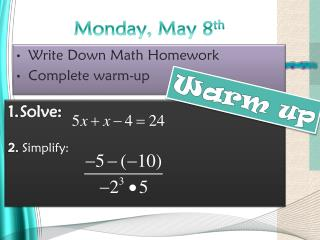# Monday, May 8 th - PowerPoint PPT PresentationDownload PresentationMonday, May 8 th

Download Presentation## Monday, May 8 th

- - - - - - - - - - - - - - - - - - - - - - - - - - - E N D - - - - - - - - - - - - - - - - - - - - - - - - - - -
##### Presentation Transcript

1. Monday, May 8th • Write Down Math Homework • Complete warm-up Warm up Solve: 2. Simplify:

2. Week at a glance Today: Math day-Box and whiskers 1 Tuesday: Science-constellations Math-Box and whiskers 2 Wednesday: Normal schedule field trip. Thursday: Review for Science Test Friday: Galaxy test Monday 5/14: MATH TEST

4. New Unit Box & Whisker

5. Part 1: Means of Central Tendency

6. Helpful Hint The mean is sometimes called the average. The mean is the sum of the data values divided by the number of data items. The median is the middle value of an odd number of data items arranged in order. For an even number of data items, the median is the average of the two middle values. The modeis the value or values that occur most often. When all the data values occur the same number of times, there is no mode. The range of a set of data is the difference between the greatest and least values. It is used to show the spread of the data in a data set.

7. Practice Problem #1 Find the mean, median, mode, and range of the data set. 4, 7, 8, 2, 1, 2, 4, 2

8. sum 8 items Mean Find the mean, median, mode, and range of the data set. 4, 7, 8, 2, 1, 2, 4, 2 mean: Add the values. 4 + 7 + 8 + 2 + 1 + 2 + 4 + 2 = 30 Divide the sum by the number of items. 30  = 3.75 8 The mean is 3.75.

9. Median Find the mean, median, mode, and range of the data set. 4, 7, 8, 2, 1, 2, 4, 2 median: Arrange the values in order. 1, 2, 2, 2, 4, 4, 7, 8 There are two middle values, so find the mean of these two values. 2 + 4 = 6 6  2 = 3 The median is 3.

10. Mode Find the mean, median, mode, and range of the data set. 4, 7, 8, 2, 1, 2, 4, 2 mode: The value 2 occurs three times. 1, 2, 2, 2, 4, 4, 7, 8 The mode is 2.

11. Range Find the mean, median, mode, and range of the data set. 4, 7, 8, 2, 1, 2, 4, 2 range: Subtract the least value 1, 2, 2, 2, 4, 4, 7, 8 from the greatest value. 8 – 1 = 7 The range is 7.

12. You Try! Use the data below for Questions 1-4. 14, 25, 37, 53, 26, 12, 70, 31 1. What is the mean? 2. What is the median? 3. What is the mode? 4. What is the range? 33.5 28.5 none 58

13. Part 2: Box and Whisker

14. Box & Whisker Plots Learn to display and analyze data in box-and-whisker plots.

15. Vocabulary box-and-whisker plot lower quartile upper quartile interquartile range

16. A box-and-whisker plot uses a number line to show the distribution of a set of data. • To make a box-and-whisker plot, first divide the data into four equal parts using quartiles. • The median, or middle quartile, divides the data into a lower half and an upper half. • The median of the lower half is the lower quartile, and the median of the upper half is the upper quartile. • The interquartile range is from the lower quartile to the upper quartile

17. Recipe • Order numbers from least to greatest • Find the Least and greatest value: WHISKERS • Find the MEDIAN: divides data in half • Find the median of the lower half: LOWER QUARTILE • Find the median of the upper half: UPPER QUARTILE • Plot all five points. MEDIANS make box

18. Caution! To find the median of a data set with an even number of values, find the mean of the two middle values. 4, 6, 8, 10 Median: 7

19. 67 67 67 67 69 69 73 73 75 75 75 75 81 81 85 85 Problem #1 Use the data to make a box-and-whisker plot. 73 67 75 81 67 75 85 69 Step 1: Order the data from least to greatest. Then find the least and greatest values, the median, and the lower and upper quartiles. The least value. The greatest value. + Find the median. 2 =74

20. 67 67 69 73 75 75 81 85 Step 1 Continued 67 + 69 lower quartile = = 68 2 75 + 81 upper quartile = = 78 2

21. Step 2: Draw a number line. Above the number line, plot points for each value in Step 1. Step 3: Draw a box from the lower to the upper quartile. Inside the box, draw a vertical line through the median. Then draw the “whiskers” from the box to the least and greatest values. 64 66 68 70 72 74 76 78 80 82 84 86

22. Classwork Reteach Box and Whisker

23. Practice, Practice, Practice Homework Practice C Box and Whisker Plots